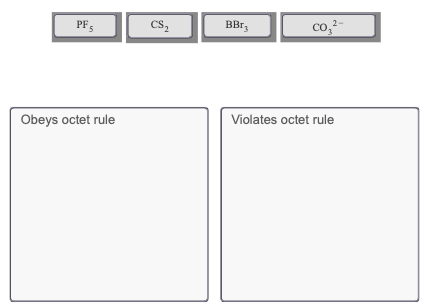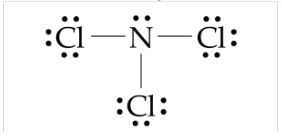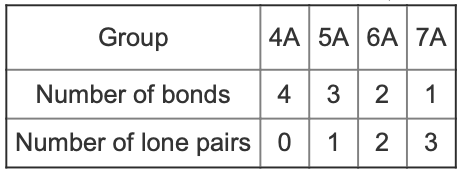# Problem: Consider the following four molecules. Which of these satisfy the octet rule and which do not?Drag the appropriate items to their respective bins.A Lewis structure shows the arrangement of atoms and valence electrons in a molecule. Most often, each bonding pair of electrons is shown as a line whereas nonbonding electrons are shown as dots as seen here. Notice that each atom is surrounded by a total of eight electrons, an octet.Octet ruleFor elements in neutral molecules, the number of bonds needed to achieve an octet follows a trend by group, as shown in the following tableExceptions to the octet ruleExceptions to the octet rule include hydrogen, which is stable with only two electrons (one bond), and boron, which is stable with only six electrons (three bonds). Additionally, elements in period 3 and beyond sometimes form more than the typical number of bonds, thus expanding their valence shells to more than eight electrons.

###### FREE Expert Solution
91% (70 ratings)View Complete Written Solution
###### Problem Details

Consider the following four molecules. Which of these satisfy the octet rule and which do not?

Drag the appropriate items to their respective bins.A Lewis structure shows the arrangement of atoms and valence electrons in a molecule. Most often, each bonding pair of electrons is shown as a line whereas nonbonding electrons are shown as dots as seen here. Notice that each atom is surrounded by a total of eight electrons, an octet.Octet rule

For elements in neutral molecules, the number of bonds needed to achieve an octet follows a trend by group, as shown in the following tableExceptions to the octet rule

Exceptions to the octet rule include hydrogen, which is stable with only two electrons (one bond), and boron, which is stable with only six electrons (three bonds). Additionally, elements in period 3 and beyond sometimes form more than the typical number of bonds, thus expanding their valence shells to more than eight electrons.